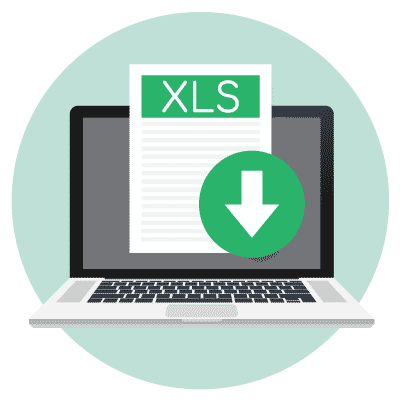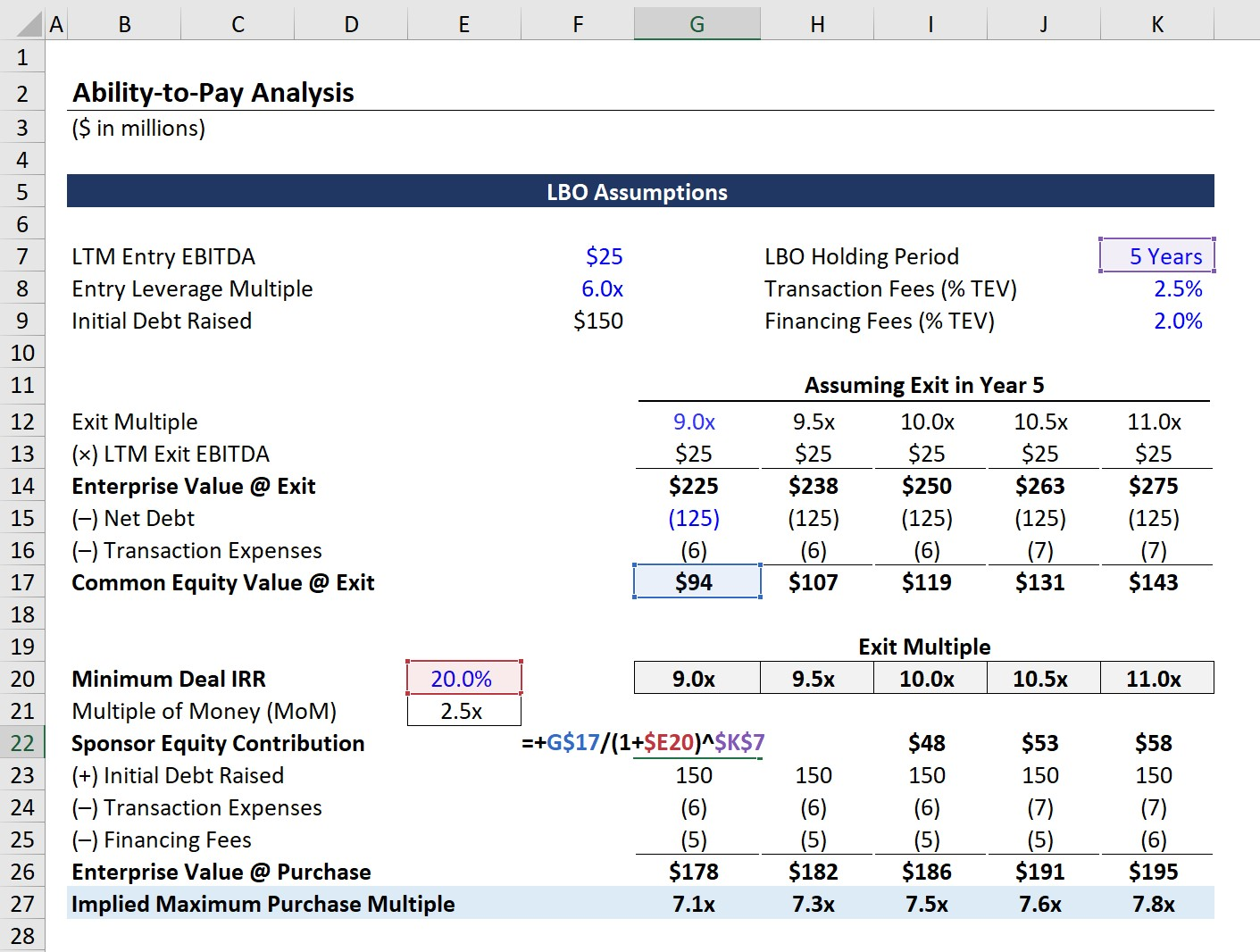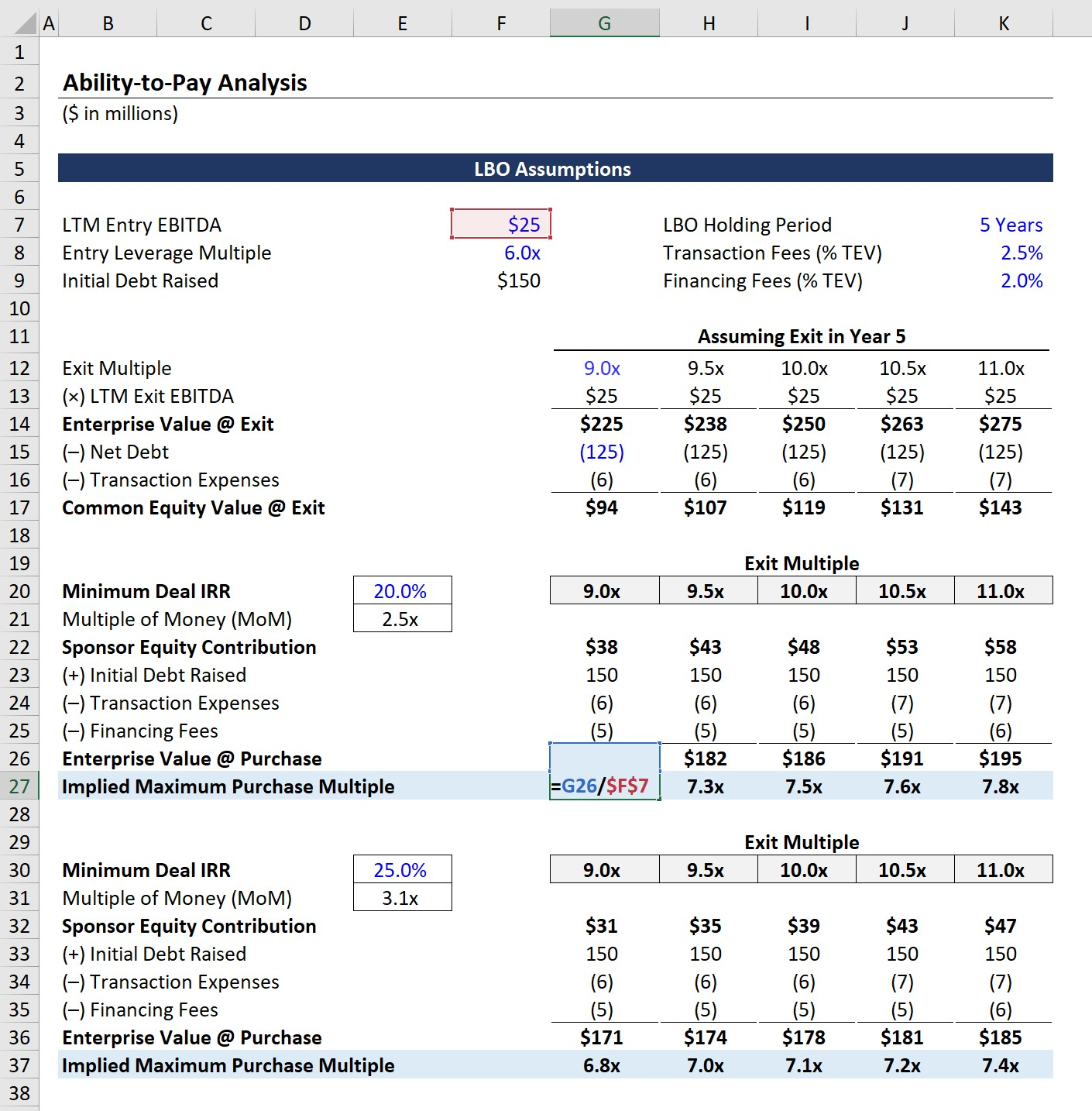# Ability-To-Pay Analysis

Learn to Perform Ability-to-Pay Analysis with Detailed Explanations of Each Calculation Step (Excel Tutorial)Key Takeaways
• How can ability-to-pay analysis be used for leveraged buyouts (LBOs)?
• Why can affordability analysis be a critical step for financial sponsors?
• In the private equity industry, what is considered to be the standard minimum internal rate of return (IRR) and multiple-of-money (MoM)?
• What is the relationship between the purchase price and LBO returns?

## Affordability Analysis in Leveraged Buyouts (LBOs)

Before we discuss how to perform an ability-to-pay analysis, we must first shed light on what a private equity firm is attempting to estimate by creating a leveraged buyout (LBO) model.

The essential question that the PE firm strives to answer is: “How much can our firm afford to approximately pay to acquire this target opportunity while still meeting our minimum return hurdles?”

When building a LBO model, one of the initial steps is to set up a basic schedule of assumptions (e.g. debt pricing, initial total debt / EBITDA ratio to fund the transaction, sponsor equity contribution).

After doing so, you can designate a range of targeted returns, or IRRs, and back into an implied purchase price (or multiple) – which is the concept behind the ability-to-pay analysis.

Once the necessary assumptions are entered, the range of purchase multiples that results in enterprise valuations that yield the targeted range of internal rate of returns (IRRs) and multiple-of-money (MoMs) can be determined.

###### IRR Hurdle Rates

A common term used in private equity is the “hurdle rate”, which refers to the minimum rate of return that financial sponsors must meet before moving forward with a LBO transaction.

Each firm will have a different hurdle rate (i.e. minimum threshold) for what is considered an “attractive” investment to actively pursue. However, the standard hurdle rate tends to range between 20% to 25%.

## LBO “Floor Valuation” Definition

At the end of the day, the projected fund returns drive investment decisions, regardless of how compelling the fundamentals of the company (and industry) are or how well the target company aligns with the fund’s portfolio strategy.

The LBO model can be used to quantify the maximum purchase price that could be paid at entry, while still realizing the minimum 20% to 25% IRR (“hurdle rate”).

Therefore, a LBO model provides a so-called “floor valuation” of a potential LBO transaction since it is used to determine what a financial sponsor could afford to pay for the target under consideration.

Given the risk profile associated with using significant debt financing and the relatively short investment horizons (~3 to 8 years), the hurdle rate to invest (i.e. the cost of equity) is going to be higher than if an identical business had no exposure to those same LBO-related risks.

Said differently, the implied present value (or target enterprise value) will be lower due to the higher hurdle rates of financial sponsors than valuations conducted under the traditional discounted cash flow (DCF) or relative valuation approaches — all else being equal.

Now that we’ve discussed the use-case of the ability-to-pay analysis for LBOs, we can proceed with an example calculation in Excel. To access the spreadsheet that goes along with our tutorial, just fill out the linked form below.Submitting ...

## LBO Model Entry Assumptions

Without further ado, let’s get started!

First, we’ll begin our modeling tutorial by listing the entry transaction assumptions:

• LTM Entry Multiple: \$25mm
• Entry Leverage Multiple: 6.0x

From those two assumptions, we can calculate that the initial amount of debt raised to finance the deal was \$150mm.

• Initial Debt Raised = \$25mm LTM EBITDA × 6.0x Leverage Multiple = \$150mm

Next, there are three more important transaction assumptions:

1. LBO Holding Period: 5 Years
2. Transaction Fees (% TEV): 2.5%
3. Financing Fees (% TEV): 2.0%## LBO Model Exit Assumptions

With those entry assumptions filled out, we can start working on the exit assumptions before reaching the ability-to-pay analysis.

For our range of potential exit multiples, the first input will be 9.0x – and using a step function of 0.5x – we’ll extend this to 11.0x.

As for remaining exit assumptions, we’ll assume the following:

• LTM Exit EBITDA = \$25mm
• Net Debt at Exit = \$125mm

To calculate the enterprise value on the date of exit, we’ll multiply the applicable exit multiple by the LTM exit EBITDA assumption.

For example, if we assume the investment will be exited at a multiple of 9.0x, the following formula is used:

• Enterprise Value @ Exit: 9.0x × \$25mm = \$225mm

Since we’re making our way down to the common equity value on the date of exit (i.e. at the end of Year 5), we must deduct the net debt and transaction expense (e.g. M&A advisory fees).

Note that the reason we deduct transaction expenses in this step, but not financing expenses, is that advisory fees are required again when selling the company, while financing fees are not.

Continuing off our 9.0x exit multiple example, the formula below calculates the residual common equity value, which we’ll recalculate for each exit multiple assumption.

• Common Equity Value @ Exit = \$225mm – \$125mm – \$6mm = \$94mm

## Ability-to-Pay Analysis Example

In the next portion of our tutorial, we can now estimate the implied purchase price to achieve our targeted deal IRR.

Here, we’ll have two target IRRs:

1. 20.0% Minimum IRR
2. 25.0% Minimum IRR

One significant component of the IRR is the required sponsor equity contribution needed to complete the transaction.

We’ll first calculate the approximate sponsor equity contribution using the formula below:

• Sponsor Equity Contribution = Common Equity Value @ Exit / (1 + Minimum Deal IRR) ^ LBO Holding PeriodUpon extrapolating this calculation for the entire range of exit multiples, we can link to our assumptions from earlier.

• (+) Initial Debt Raised: \$150mm
• (–) Transaction Expenses: TEV × Transaction Fees %
• (–) Financing Fees: TEV × Financing Fees %

If you are wondering why transaction fees are accounted for twice, this is because M&A advisory services are required two times:

2. Exiting the Investment (Sell-Side M&A)

Once completed, we have the enterprise value as of the date of purchase. From the enterprise value at exit, we can divide that figure by the LTM entry multiple to get the implied purchase multiple that enables us to achieve the desired return.

• Implied Maximum Purchase Multiple = Enterprise Value @ Exit ÷ LTM Entry EBITDAAccording to our model, assuming an exit multiple of 10.0x and required minimum IRR of 20.0x, the maximum purchase multiple we should be willing to pay should be around ~7.5x.

The same steps from our 20.0% hurdle rate section could subsequently be replicated for the 25.0% minimum deal IRR, as well as for higher required IRRs if needed.

###### Side Note: Accuracy of Implied Multiples

Keep in mind, however, that the ability-to-pay analysis is only an approximation of the maximum purchase price (and multiple) and there remains room for error.

For example, to keep our model simplistic and avoid the circularity, we used the TEV on the date of exit rather than entry when calculating the transaction fees.

While these minor discrepancies are unlikely to have a tangible impact on the pricing recommendation, these imperfections can cause slight mismatches in the return figures and are something to be aware of.

Hence, most complex LBO models with ATP tabs use the “ROUND” Excel function, as the implied multiples are meant to serve as a rough point of reference (i.e. an estimated ceiling), but NOT a precise purchase multiple.

To recap, a LBO model is often called a “floor valuation” as it can be used to determine the maximum purchase price the buyer can pay while still reaching the fund specific returns thresholds.

The purchase multiple is among the most important factors that determine the success (or failure) of a LBO — which an ability-to-pay analysis can help guide.Inline FeedbacksLearn Financial Modeling Online

Everything you need to master financial and valuation modeling: 3-Statement Modeling, DCF, Comps, M&A and LBO.

X

The Wall Street Prep Quicklesson Series

7 Free Financial Modeling Lessons

Get instant access to video lessons taught by experienced investment bankers. Learn financial statement modeling, DCF, M&A, LBO, Comps and Excel shortcuts.